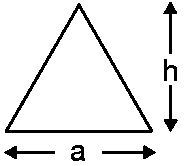# Equilateral Triangle Formula

As the name suggests, ‘equi’ means Equal, an equilateral triangle is the one where all sides are equal and have an equal angle. The internal angles of the equilateral triangle are also the same, that is, 60 degrees. Here are the formulas for area, altitude, perimeter, and semi-perimeter of an equilateral triangle.Where,
a is the side of an equilateral triangle.
h is the altitude of an equilateral triangle.

#### Equilateral Triangle Formulas

$$\begin{array}{l}\large Area \;of \;an \;Equilateral \;Triangle = \frac{\sqrt{3}}{4}a^{2}\end{array}$$

$$\begin{array}{l}\large Perimeter \;of \;an \;Equilateral \;Triangle = 3a\end{array}$$

$$\begin{array}{l}\large Semi \;Perimeter \;of \;an \;Equilateral \;Triangle = \frac{3a}{2}\end{array}$$

$$\begin{array}{l}\large Height \;of \;an \;Equilateral \;Triangle = \frac{\sqrt{3}}{2}a\end{array}$$

### Solved Example

Question: Find the area, altitude, perimeter and semi-perimeter of an equilateral triangle whose side is 8 cm.

Solution:

Side of an equilateral triangle = a = 8 cm

Area of an equilateral triangle

=

$$\begin{array}{l}\frac{\sqrt{3}}{4}\end{array}$$
$$\begin{array}{l}a^{2}\end{array}$$

=

$$\begin{array}{l}\frac{\sqrt{3}}{4}\end{array}$$

$$\begin{array}{l}\times\end{array}$$
$$\begin{array}{l}8^{2}\end{array}$$
cm2

=

$$\begin{array}{l}\frac{\sqrt{3}}{4}\end{array}$$

$$\begin{array}{l}\times\end{array}$$
64 cm2

= 21.712 cm2

Altitude of an equilateral triangle

=

$$\begin{array}{l}\frac{\sqrt{3}}{2}\end{array}$$
a

=

$$\begin{array}{l}\frac{\sqrt{3}}{2}\end{array}$$

$$\begin{array}{l}\times\end{array}$$
8 cm

= 6.928 cm

Perimeter of an equilateral triangle

= 3a

= 3

$$\begin{array}{l}\times\end{array}$$
8 cm

= 24 cm

Semi-Perimeter of an equilateral triangle

=

$$\begin{array}{l}\frac{3a}{2}\end{array}$$

=

$$\begin{array}{l}\frac{3\times 8}{2}\end{array}$$
cm

=

$$\begin{array}{l}\frac{24}{2}\end{array}$$
cm

= 12 cm